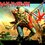# Indian Regional Mathematical Olympiad 2013(problem 2)

If $a$,$b$ and $c$ are positive integers such that $a | b^{5}$ , $b|c^{5}$ and $c|a^{5}$.Prove that $abc|(a+b+c)^{31}$.7 years, 3 months ago

This discussion board is a place to discuss our Daily Challenges and the math and science related to those challenges. Explanations are more than just a solution — they should explain the steps and thinking strategies that you used to obtain the solution. Comments should further the discussion of math and science.

When posting on Brilliant:

• Use the emojis to react to an explanation, whether you're congratulating a job well done , or just really confused .
• Ask specific questions about the challenge or the steps in somebody's explanation. Well-posed questions can add a lot to the discussion, but posting "I don't understand!" doesn't help anyone.
• Try to contribute something new to the discussion, whether it is an extension, generalization or other idea related to the challenge.

MarkdownAppears as
*italics* or _italics_ italics
**bold** or __bold__ bold
- bulleted- list
• bulleted
• list
1. numbered2. list
1. numbered
2. list
Note: you must add a full line of space before and after lists for them to show up correctly
paragraph 1paragraph 2

paragraph 1

paragraph 2

[example link](https://brilliant.org)example link
> This is a quote
This is a quote
    # I indented these lines
# 4 spaces, and now they show
# up as a code block.

print "hello world"
# I indented these lines
# 4 spaces, and now they show
# up as a code block.

print "hello world"
MathAppears as
Remember to wrap math in $$ ... $$ or $ ... $ to ensure proper formatting.
2 \times 3 $2 \times 3$
2^{34} $2^{34}$
a_{i-1} $a_{i-1}$
\frac{2}{3} $\frac{2}{3}$
\sqrt{2} $\sqrt{2}$
\sum_{i=1}^3 $\sum_{i=1}^3$
\sin \theta $\sin \theta$
\boxed{123} $\boxed{123}$

Sort by:

in general if $a | b^n$ , $b | c^n$ , $c | a^n$ , $abc | (a+b+c)^{n^2 + n + 1}$

- 7 years, 3 months ago

Here a , b, c are becoming equal no?

- 7 years, 3 months ago

$a,b,c$ are not necessarily equal, if that's what you mean.

- 7 years, 3 months ago

You re correct ...at first glance it looked like that....:p I sometimes suffer from brain malfunctions like these.....

- 7 years, 3 months ago

This was my favorite question of the set. It looks so elegant, and is simple to approach if you are careful with the details.

Staff - 7 years, 3 months ago

Outline:

Consider each term of the expansion of $(a+b+c)^{31},$ which is of the form $m a^{p} b^{q} c^{r},$ where $m, a, b, r \in \mathbb{Z^+}$ and $p+q+r=31.$ If $p,q,r>0,$ $abc \mid a^p b^q c^r.$ You just need to handle the exceptional cases when atleast one of $p,q,r$ is zero, which is not hard. I'll post my full solution if necessary.

I got this problem when I sat for RMO last time. Heaven knows why I couldn't solve it in the examination hall... it was pretty easy.

- 7 years, 3 months ago

I solved this problem orally with you while coming down the staircase :P

- 7 years, 3 months ago

Yep, I remember! :P I couldn't solve it in the hall, though.

- 7 years, 3 months ago

This seems fairly easy for an Olympiad problem

- 6 years, 9 months ago